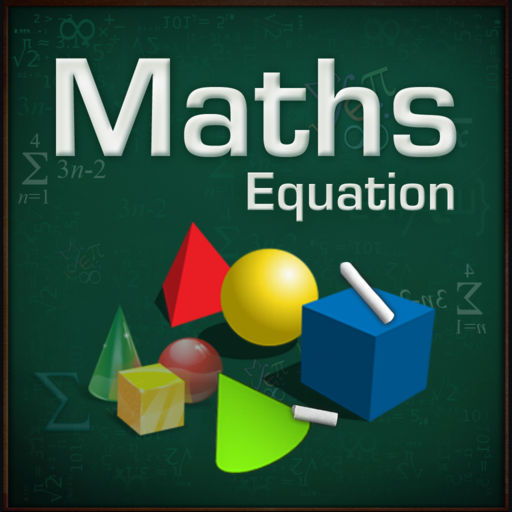Maths Equation# Maths Equation

## Tatvasoft

1.1 for iPhone
Free
0

0 Ratings

Update Date

2013-01-08

Size

10.9 MB

Maths Equation Description
Maths Equation is a very fast and easy tool to solve quadratic equations and find area of area of 6 shapes. You input your numbers and instantaneously get solution in Fractions of second.

Excellent app for students, teachers, scientists and engineers who need an immediate and easy verification of their quadratic equations solution and area of shapes.

This app can calculate the area of 6 SHAPES! With these 6 shapes, you can find the area of almost any shape.

Features
★Equations to Support
- linear equation (ax+b=c)
- cubic equation (ax^3+bx^2+cx+d=0)
- 2 unknowns (x,y)
- 3 unknowns (x,y,z)

★Geometric Figures
- Find area of triangle
- Find area of square
- Find area of circle
- Find area of polygon
- Find area of cylinder
- Find area of cone
Maths Equation 1.1 Update
2013-01-08 Version History
Minor Change
More